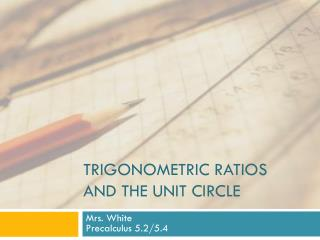DownloadDownload PresentationTrigonometric ratios and the unit circle

# Trigonometric ratios and the unit circle

Download Presentation## Trigonometric ratios and the unit circle

- - - - - - - - - - - - - - - - - - - - - - - - - - - E N D - - - - - - - - - - - - - - - - - - - - - - - - - - -
##### Presentation Transcript

1. Trigonometric ratios and the unit circle Mrs. WhitePrecalculus 5.2/5.4

2. Schedule

3. Trig Ratios and the Unit Circle • Goals • Students should be able to calculate the exact value of the 6 trigonometric ratios.

4. Review – Complete the Table

5. Example 1 • Plot P (-12, -5) in the coordinate plane. If the terminal side of an angle in standard position passes through P, find the 6 trig. Ratios.

6. Example 2 • Plot P (5, -2) in the coordinate plane. If the terminal side of an angle in standard position passes through P, find the 6 trig. Ratios.

7. Example 3 • Given the following, find the 6 trigonometric ratios: • sec Θ = -2 • sin Θ > 0

8. Example 4 • Find the reference angle, α. • Given: • Θ = 309o • α = 360o - 309o • α = 51o • Find the reference angle, α. • Given: • Θ = • Θ = • α =

9. Example 5 • Evaluate the 6 trig functions of the following angles. No Calculator. • Θ = 510° • This is coterminal with: 150° • Reference angle: 30°, Quadrant 2

10. Example 6 • Evaluate the 6 trig functions of the following angles. No Calculator. • Θ = • This is coterminal with: • Reference angle: , Quadrant 3

11. Example 7 • Sketch a right triangle corresponding to the trig function of the acute angle, Θ. Find the length of the missing side (using Pythagorean Th). Evaluate the remaining 5 trig. functions at Θ. • Given: Θ